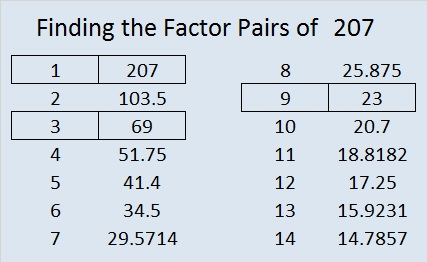# 207 and Level 1

• 207 is a composite number.
• Prime factorization: 207 = 3 x 3 x 23, which can be written 207 = 3² x 23
• The exponents in the prime factorization are 2 and 1. Adding one to each and multiplying we get (2 + 1)(1 + 1) = 3 x 2  = 6. Therefore 207 has exactly 6 factors.
• Factors of 207: 1, 3, 9, 23, 69, 207
• Factor pairs: 207 = 1 x 207, 3 x 69, or 9 x 23
• Taking the factor pair with the largest square number factor, we get √207 = (√9)(√23) = 3√23 ≈ 14.38749——————————————————————

Excel file of puzzles and previous week’s factor solutions: 10 Factors 2014-08-18This site uses Akismet to reduce spam. Learn how your comment data is processed.